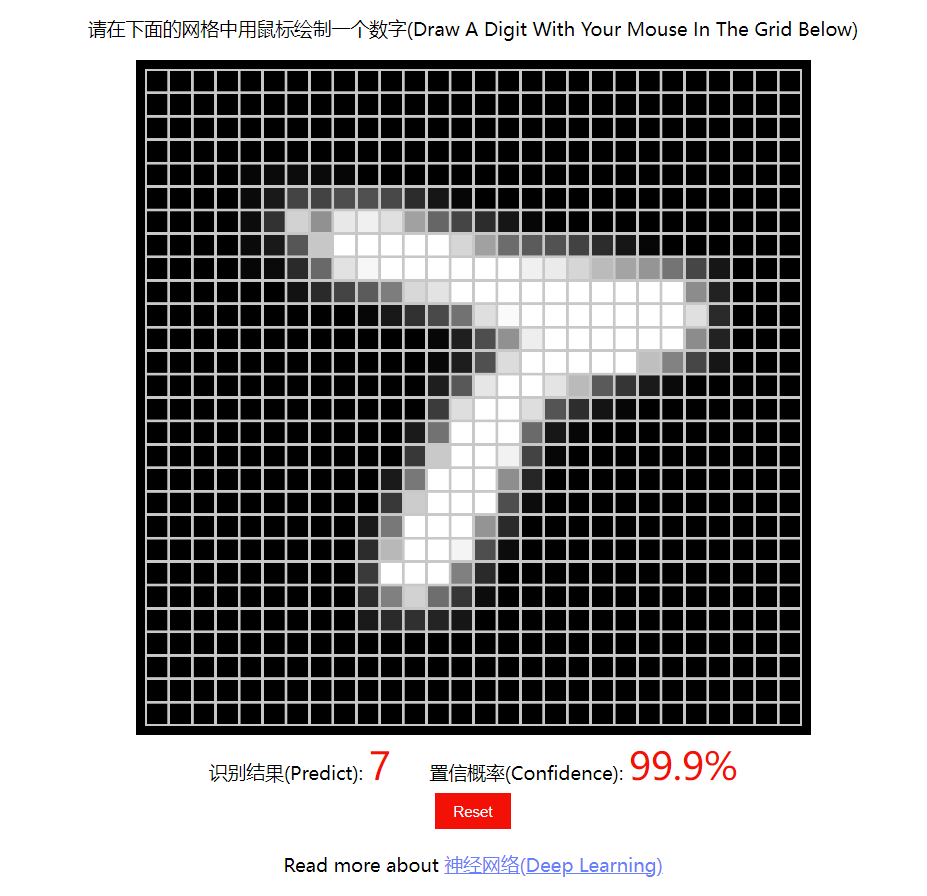By Long Luo

# 神经网络是如何工作的？

$S(x) = {\frac {1}{1 + e^{-x}}} = {\frac {e^{x}}{e^{x} + 1}} = 1 - S(-x)$

# 手写数字识别https://ml5js.org/